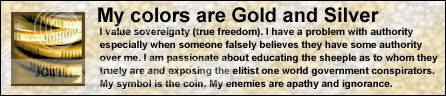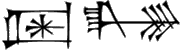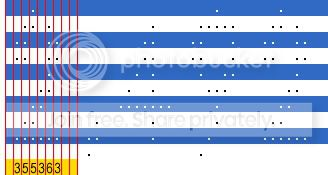## Recommended Posts

I need a way to convert the "." in the attach file into numbers. Right now i have a program that looks at the screen for a certain color and return every where it is and isn't. It returns the file attached. but i need a way to make it see them as numbers and not just "." I dont need anyone to write the code, I wouldn't expect you to, I just need some help coming up with a way. One way I was thinking was If i could separate all of the digits into its own group i could just count the number of "." and the number of "." would correspond to a number. But i don't know how i would separate the digits.

Any Ideas?

Thanks

Example.txt

Edited by narayanjr

##### Share on other sites

One way would be to load it into an array and figure it out from there.

Agreement is not necessary - thinking for one's self is!##### Share on other sites

I guess Im just not seeing this, but what would I do with it after that?

And just in case you cant read it, the numbers are

2,492,671,933

And the numbers change every so often so I can just have it read a certain number of characters from each line, because they will always change.

##### Share on other sites

If you aren't just a nerd with lots of time on his hands - what will this conversion help you to, what do you get out of it.

One way could proceed, if you absolutely need the result such as the string "2,492,671,933" is to do a screen capture, stretch the resulting image file to make it about 8 percent of its original size, and use an optical character reader. Good OCR's are expensive and use a lot of memory, but for this, the OCR wouldn't have to run for very long.

##### Share on other sites

Its for a game, Its reading numbers on the screen and I need to convert the "." into numbers so I can do some Math operations to it and get a new number. And I dont really want to use an OCR. Dont want to spend money on it and I need this to work on many different computers.

Edited by narayanjr

##### Share on other sites

Are the "." (dots) actually text or are they a graphic?

Agreement is not necessary - thinking for one's self is!##### Share on other sites

They are Text. The File I attached is the Exact file that the program returns. That is the final product of the program right now.

##### Share on other sites

Its for a game, Its reading numbers on the screen and I need to convert the "." into numbers so I can do some Math operations to it and get a new number. And I dont really want to use an OCR. Dont want to spend money on it and I need this to work on many different computers.

If dot-numbers always look the same, there is a way to do this the way you want it done. You might start by coming up with definitions of each of the numbers that look like this:

Definition of a seven in position number 1 -

Line 1 - 10 dots starting at character 7 extending thru character 16

Line 2 - 8 dots starting at character 5 extending thru character 14

etcetera ...

Line 22 - the final line - 3 dots starting at character 5 extending thru character 7, double-spaced.

Then you would need to account for the dot-number being in a certain position within the total dot-number string.

Lastly, you would need to account for the presence of those dot-commas, which are narrower that the dot-numbers.

You will probably decide to do something more productive with your time. This would be very tedious work that will probably drive you mad before you have finished the project in about 3 weeks time.

Edited by Squirrely1

##### Share on other sites

Look closely at this example.You should see a pattern that you can load into an array to determine the text. It's very similar to an OCR technique used here.

It should be fairly easy to figure out once you comprehend the technique. 10 lines of data, 94 characters per line, so an array might look like \$aArray.

I'm watching a movie right now, so you'll have to figure out the rest. I'll help out when I see a good effort put forth.

Edited by Fossil Rock

Agreement is not necessary - thinking for one's self is!##### Share on other sites

Well it wasnt that hard once i figured out a way. I put each character into an array. Then had it go through and find every digit by finding all of the "." in between 2 blank columns. Then i just had it count the number of dots. The number of dots corresponds to the value of the digit. If two or more Digits have the same number off dots then i made it read half of the digit and went off of those dots.

Thanks for the help

##### Share on other sites

Well it wasnt that hard once i figured out a way. I put each character into an array. Then had it go through and find every digit by finding all of the "." in between 2 blank columns. Then i just had it count the number of dots. The number of dots corresponds to the value of the digit. If two or more Digits have the same number off dots then i made it read half of the digit and went off of those dots.

Thanks for the help

Care to share your code for others to learn from or possibly improve upon? Edited by Fossil Rock

Agreement is not necessary - thinking for one's self is!##### Share on other sites

Well by no means is it neat or organized but here it is. If you can follow the code more power to you. Any questions just ask and ill tell you what it does. If any one can redo any of it to make it shorter that would be nice but Im not expecting any thing. Good luck following the code.

```Opt("WinTitleMatchMode", 2)
Opt("TrayIconDebug", 1)
Opt("PixelCoordMode", 2)
Opt("MouseCoordMode", 2)
#include <Array.au3>

Global \$StartPosX, \$StartPosY, \$EndPosX, \$EndPosY, \$SecondC, \$D
Dim \$DigitValue
\$D = 0
\$White = 12895428

\$CurrentXp = FileOpen("Current Xp.txt", 2 )
\$NextXp = FileOpen("Next Xp.txt", 2 )

;TopLeft(153,107)
;BottomRight(254,117)
\$StartCurrentPosX = 153
\$StartCurrentPosY = 108
\$EndCurrentPosX = 254
\$EndCurrentPosY = 117

\$StartNextPosX = 284
\$StartNextPosY = 108
\$EndNextPosX = 377
\$EndNextPosY = 117

For \$Y = \$StartCurrentPosY to \$EndCurrentPosY
For \$X = \$StartCurrentPosX To \$EndCurrentPosX
If PixelGetColor(\$X, \$Y) = \$White Then
FileWrite(\$CurrentXp, ".")
Else
FileWrite(\$CurrentXp, " ")
EndIf
Next
FileWrite(\$CurrentXp, @CRLF)
Next

For \$Y = \$StartNextPosY to \$EndNextPosY
For \$X = \$StartNextPosX To \$EndNextPosX
If PixelGetColor(\$X, \$Y) = \$White Then
FileWrite(\$NextXp, ".")
Else
FileWrite(\$NextXp, " ")
EndIf
Next
FileWrite(\$NextXp, @CRLF)
Next
FileClose(\$CurrentXp)
FileClose(\$NextXp)

\$CurrentXp = FileOpen("Current Xp.txt", 0)
Dim \$CurXpArray
\$r = 0
While 1
If @error = -1 Then ExitLoop
For \$C = 0 To StringLen(\$CurrentXpLine)
\$Char = StringMid(\$CurrentXpLine, \$C+1, 1)
\$CurXpArray[\$r][\$C] = \$Char
Next
\$r = \$r + 1
WEnd

\$SecondC = 0
\$FirstDot = 0
\$F = 0
\$F2 = 0
For \$C2 = 0 to UBound(\$CurXpArray,2) - 1
\$ColumnCount = 0
\$SecondC = \$SecondC + 1
For \$R2 = 0 to UBound(\$CurXpArray,1) - 1
If \$CurXpArray[\$R2][\$C2] = " " Then
\$ColumnCount = \$ColumnCount + 1
ContinueLoop
ElseIf \$CurXpArray[\$R2][\$C2] = "." Then
\$FirstDot = 1
ExitLoop
EndIf
If \$ColumnCount = 10 And \$FirstDot = 1 Then
\$F2 = \$F
\$F = \$SecondC - 1
\$DigitFile = FileOpen("Digit.txt",2)
For \$DR = 0 to UBound(\$CurXpArray,1) - 1
For \$DC = \$F2 to \$F
FileWrite(\$DigitFile, \$CurXpArray[\$DR][\$DC])
Next
FileWrite(\$DigitFile, @CRLF)
Next
\$ColumnCount = 0
FileClose(\$DigitFile)
;MsgBox(0,"","Pause to look")
GetRidOfWhite()
\$FirstDot = 0
;Exit
EndIf
Next
Next
\$CurrentXpValue =0
For \$V = 0 To UBound(\$DigitValue) -1
If \$DigitValue[\$V] <> 4 Then
If \$DigitValue[\$V] = 35 Then
\$XpDigitValue = 0
ElseIf \$DigitValue[\$V] = 9 Then
\$XpDigitValue = 1
ElseIf \$DigitValue[\$V] = 13 Then
\$XpDigitValue = 2
ElseIf \$DigitValue[\$V] = 24 Then
\$XpDigitValue = 3
ElseIf \$DigitValue[\$V] = 18 Then
\$XpDigitValue = 4
ElseIf \$DigitValue[\$V] = 15 Then
\$XpDigitValue = 5
ElseIf \$DigitValue[\$V] = 12 Then
\$XpDigitValue = 6
ElseIf \$DigitValue[\$V] = 14 Then
\$XpDigitValue = 7
ElseIf \$DigitValue[\$V] = 29 Then
\$XpDigitValue = 8
ElseIf \$DigitValue[\$V] = 7 Then
\$XpDigitValue = 9
EndIf

\$CurrentXpValue = \$CurrentXpValue & \$XpDigitValue
EndIf
Next
\$RealCurrentXpValue = Number(\$CurrentXpValue)
MsgBox(0,"",\$RealCurrentXpValue)

Func GetRidOfWhite()
;MsgBox(0,"","Rid of White")
\$DigitFile = FileOpen("Digit.txt",0)
\$NoWhiteDigitFile = FileOpen("No White Digit.txt",2)
While 1
If @error = -1 Then ExitLoop
\$NewLine = StringReplace(\$line, " ","")
FileWriteLine(\$NoWhiteDigitFile, \$NewLine)
Wend
FileClose(\$NoWhiteDigitFile)
FileClose(\$DigitFile)
;MsgBox(0,"","Pause to look")
GetTotalDots()
EndFunc

Func GetTotalDots()
;MsgBox(0,"","count dots")
\$NoWhiteDigitFile = FileOpen("No White Digit.txt",0)
\$TotalDots = 0

While 1
If @error = -1 Then ExitLoop
For \$C = 0 To StringLen(\$NoWhiteDigitFileLine)
\$Char = StringMid(\$NoWhiteDigitFileLine, \$C+1, 1)
;MsgBox(0,"",\$Char)
If \$Char = "." Then
\$TotalDots = \$TotalDots + 1
EndIf
Next
WEnd
FileClose(\$NoWhiteDigitFile)
If \$TotalDots = 24 Then
\$NoWhiteDigitFile = FileOpen("No White Digit.txt",0)
\$TotalDots = 0
\$LineNumber = 1
While \$LineNumber < 6
If @error = -1 Then ExitLoop
For \$C = 0 To StringLen(\$NoWhiteDigitFileLine)
\$Char = StringMid(\$NoWhiteDigitFileLine, \$C+1, 1)
;MsgBox(0,"",\$Char)
If \$Char = "." Then
\$TotalDots = \$TotalDots + 1
EndIf
Next
\$LineNumber = \$LineNumber + 1
WEnd
FileClose(\$NoWhiteDigitFile)
ElseIf \$TotalDots = 22 Then
\$NoWhiteDigitFile = FileOpen("No White Digit.txt",0)
\$TotalDots = 0
\$LineNumber = 1
While \$LineNumber < 7
If @error = -1 Then ExitLoop
For \$C = 0 To StringLen(\$NoWhiteDigitFileLine)
\$Char = StringMid(\$NoWhiteDigitFileLine, \$C+1, 1)
;MsgBox(0,"",\$Char)
If \$Char = "." Then
\$TotalDots = \$TotalDots + 1
EndIf
Next
\$LineNumber = \$LineNumber + 1
WEnd
If \$TotalDots = 18 Then
\$TotalDots = 7
EndIf
FileClose(\$NoWhiteDigitFile)
EndIf
;MsgBox(0,"",\$TotalDots)
;MsgBox(0,"","First")

\$DigitValue[\$D] = \$TotalDots
\$D = \$D + 1

EndFunc

Dim \$DigitValue
\$NextXp = FileOpen("Next Xp.txt", 0)
Dim \$CurXpArray
\$r = 0
\$D = 0
While 1
If @error = -1 Then ExitLoop
For \$C = 0 To StringLen(\$NextXpLine)
\$Char = StringMid(\$NextXpLine, \$C+1, 1)
\$CurXpArray[\$r][\$C] = \$Char
Next
\$r = \$r + 1
WEnd

\$SecondC = 0
\$FirstDot = 0
\$F = 0
\$F2 = 0
For \$C2 = 0 to UBound(\$CurXpArray,2) - 1
\$ColumnCount = 0
\$SecondC = \$SecondC + 1
For \$R2 = 0 to UBound(\$CurXpArray,1) - 1
If \$CurXpArray[\$R2][\$C2] = " " Then
\$ColumnCount = \$ColumnCount + 1
ContinueLoop
ElseIf \$CurXpArray[\$R2][\$C2] = "." Then
\$FirstDot = 1
ExitLoop
EndIf
If \$ColumnCount = 10 And \$FirstDot = 1 Then
\$F2 = \$F
\$F = \$SecondC - 1
\$DigitFile = FileOpen("Digit.txt",2)
For \$DR = 0 to UBound(\$CurXpArray,1) - 1
For \$DC = \$F2 to \$F
FileWrite(\$DigitFile, \$CurXpArray[\$DR][\$DC])
Next
FileWrite(\$DigitFile, @CRLF)
Next
\$ColumnCount = 0
FileClose(\$DigitFile)
;MsgBox(0,"","Pause to look")
GetRidOfWhite()
\$FirstDot = 0
;Exit
EndIf
Next
Next
\$CurrentXpValue =0
For \$V = 0 To UBound(\$DigitValue) -1
If \$DigitValue[\$V] <> 4 Then
If \$DigitValue[\$V] = 35 Then
\$XpDigitValue = 0
ElseIf \$DigitValue[\$V] = 9 Then
\$XpDigitValue = 1
ElseIf \$DigitValue[\$V] = 13 Then
\$XpDigitValue = 2
ElseIf \$DigitValue[\$V] = 24 Then
\$XpDigitValue = 3
ElseIf \$DigitValue[\$V] = 18 Then
\$XpDigitValue = 4
ElseIf \$DigitValue[\$V] = 15 Then
\$XpDigitValue = 5
ElseIf \$DigitValue[\$V] = 12 Then
\$XpDigitValue = 6
ElseIf \$DigitValue[\$V] = 14 Then
\$XpDigitValue = 7
ElseIf \$DigitValue[\$V] = 29 Then
\$XpDigitValue = 8
ElseIf \$DigitValue[\$V] = 7 Then
\$XpDigitValue = 9
EndIf

\$CurrentXpValue = \$CurrentXpValue & \$XpDigitValue
EndIf
Next
\$RealCurrentXpValue = Number(\$CurrentXpValue)
MsgBox(0,"",\$RealCurrentXpValue)```
Edited by narayanjr

## Create an account

Register a new account

• ### Recently Browsing   0 members

×

• Wiki

• Back

• #### Beta

• Git
• FAQ
• Our Picks
×
• Create New...